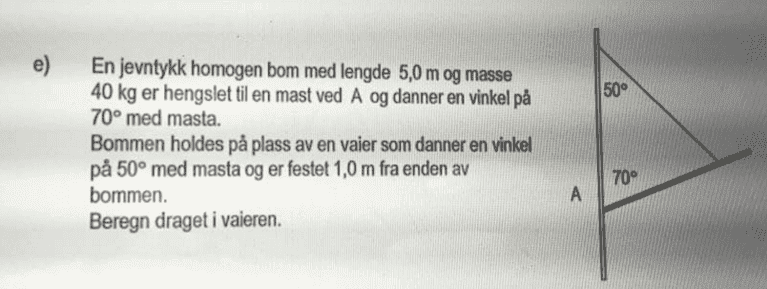# Tension of rope

## Homework StatementI'm sorry, I'm not the best in English, but I'll try to translate it.
A homogenous rod with length 5.0 m and mass 40 kg, is connected to a pole at 70* at point A.
The rod is held in place with a rope that makes 50* with the pole, and is connected to the rod 1.0 m from the end of the rod.
Determine the tension in the rope.

ΣF=0, ΣM=0

## The Attempt at a Solution

We are four people with four different answers... No one knows what anyone does wrong.. Here are two of the attempts:

https://scontent-frt3-1.xx.fbcdn.net/v/t34.0-12/13336307_10153546169760759_1312148898_n.jpg?oh=a90f8bf982bd9246081fd2d8bb251203&oe=57506221

Buzz Bloom
Gold Member
I would like to make some suggestions, but without labels on the diagram, it becomes awkward to do this.
In the diagram, label he point where the rope attaches to the rod as B.
Label the center of gravity of the rod as C.
What is the downward force FC at point C?
What is the downward force FB at point B?
What is the component of FB in the direction that stretches the rope?

Hope this helps.

Regards,
Buzz

I would like to make some suggestions, but without labels on the diagram, it becomes awkward to do this.
In the diagram, label he point where the rope attaches to the rod as B.
Label the center of gravity of the rod as C.
What is the downward force FC at point C?
What is the downward force FB at point B?
What is the component of FB in the direction that stretches the rope?

We have denoted FC as 40 * 9.81 N = 392 N, just simplifying the distributed mass as an average in the middle.
But this would be the total downward force for the entire rod, so that would mean no other downward force for the rest of the rod, including in FB. I get a little confused of this.

haruspex
Homework Helper
Gold Member
We have denoted FC as 40 * 9.81 N = 392 N, just simplifying the distributed mass as an average in the middle.
But this would be the total downward force for the entire rod, so that would mean no other downward force for the rest of the rod, including in FB. I get a little confused of this.
That's all valid.
Of the two images you attached, I cannot follow the logic in the first one.
The second method is correct and obtains the right answer.

•Norway
Buzz Bloom
Gold Member
Hi Norway:

I apologize for being careless with my use of language.
The rod is not moving. The left end of the rod is held fixed by its touching the vertical post. If the rope was not attached the rod would fall, rotating clockwise.
The tension in the rope at B exerts an angular upward force in the direction along the rope. This force balances the force FC so that the rod does not rotate.

The clockwise torque on the rod at C balances the counter-clockwise torque at B.

I hope this helps better.

Regards,
Buzz

•Norway
Thank you both very much! We got it now :-)